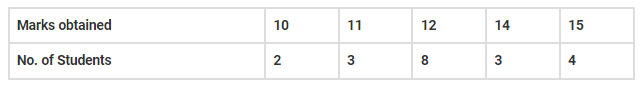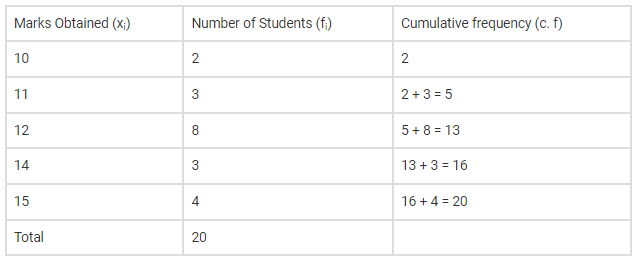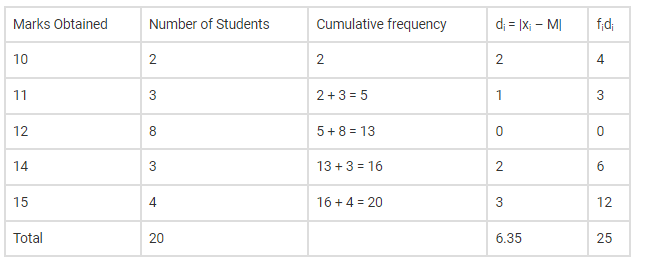# Find the mean deviation about the median`
Question:

Find the mean deviation about the median of the following distribution:Solution:

Given data distribution

Now we have to find the mean deviation about the median

Let us make a table of the given data and append other columns after calculationsNow, here N=20, which is even.

Here median,

$M=\frac{1}{2}\left[\left(\frac{N}{2}\right)^{\text {th }}\right.$ observation $+\left(\frac{N}{2}+1\right)^{\text {th }}$ observation $]$

$M=\frac{1}{2}\left[\left(\frac{20}{2}\right)^{\text {th }}\right.$ observation $+\left(\frac{20}{2}+1\right)^{\text {th }}$ observation $]$

$M=\frac{1}{2}\left[10^{\text {th }}\right.$ observation $+11^{\text {th }}$ observation $]$

Both these observations lie in cumulative frequency 13, for which corresponding observation is 12 .

$M=\frac{1}{2}[12+12]=12$

So the above table with more columns is as shown below,Hence Mean Deviation becomes,

M. D $=\frac{\sum f_{\mathrm{i}} \mathrm{d}_{\mathrm{i}}}{\sum f_{\mathrm{i}}}=\frac{25}{20}=1.25$

Therefore, the mean deviation about the median of the distribution is 1.25